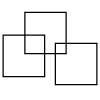#### You may also like### Three Squares

What is the greatest number of squares you can make by overlapping three squares?### Two Dice

Find all the numbers that can be made by adding the dots on two dice.### Biscuit Decorations

Andrew decorated 20 biscuits to take to a party. He lined them up and put icing on every second biscuit and different decorations on other biscuits. How many biscuits weren't decorated?

# How Do You See It?

##### Age 5 to 11Challenge Level

We had a number of solutions sent in that provided the "answers" to the calculations. Voltaire from Ravenor Primary School in England sent in the calculations explanation as follows:

$1.5$ doing $3+2=5$, $2.5$ doing $8-3=5$ $3.3$ doing $9-6=3$,

$4.3$ doing $8-5=3$, $5.6$ doing $9-3=6$, $6.10$ doing $3+7=10$

Our first contribution to the Primary site, not in English came from Jose at the Esapana Istituto;

Esto es para dos de los problemas. Mi hermana pequeña, Anya ellos lo hicieron por mÃ­. Ella tiene cinco años.
Zoe tiene 3 caramelos. Rajneeti le da dos más. 3 +2 = 5 / tres y dos son cinco
Karala tena 8 muñecas.
Ella le dio tres de ellos a su hermana pequeña. ¿Cómo ha Karala muchas muñecas tiene ahora?
As que 8-3 = 5 / ocho menos tres es igual a cinco

Which I think says:

This is for two problems. My little sister, Anya they did for me. She is five years.

Zoe has $3$ candies. Rajneeti gives you two more. $3 +2 = 5$ / three and two are
five

Kerala had $8$ dolls.
She gave three of them to his little sister. How many has Karala
dolls have now?

So $8-3 = 5$ / eight minus three is equal to five

Thank you Jose, for that. Thanks also to Voltaire